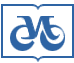## Publishing House SB RAS:

Address of the Publishing House SB RAS:
Morskoy pr. 2, 630090 Novosibirsk, Russia

# Home – Home – Jornals – Russian Geology and Geophysics 2003 number 10

## Russian Geology and Geophysics

### 2003 year, number 10

#### PROPAGATION AND ATTENUATION OF ELECTROMAGNETIC WAVES IN INHOMOGENEOUS MEDIA: NUMERICAL MODELING USING LAGUERRE TRANSFORM

A.F. Mastryukov and B.G. Mikhailenko
Institute of Computing Mathematics and Mathematical Geophysics, Siberian Branch of the RAS,
6 prosp. Lavrentieva, Novosibirsk, 630090, Russia
Keywords: Maxwell's equations, electromagnetic waves, electric field, magnetic field, relaxation time, electrical conductivity, dielectric permittivity, finite-difference solution, Laguerre transform
Pages: 1026-1034

Abstract

Maxwell's equations simulating 1D wave propagation in frequency-dependent media are derived using a robust and computationally efficient algorithm of the Laguerre transform approximation of fourth-order accuracy in space. Maxwell's equations reduce to a harmonic series of linear algebraic equations, and the convolution of the relaxation functions reduces to simple superposition of the harmonics. The equations have a single inverse matrix for all harmonics and each harmonic is obtained by multiplication of this matrix by the right term.
With a proper choice of modeling parameters, the Laguerre scheme is superior to many FDTD codes as it provides a better than second-order accurate approximation in time and shows a slower error accumulation. Compared to the Fourier transform, the Laguerre solution is as accurate but times less expensive.
The new algorithm is applicable without significant limitations to Maxwell's equations for two- and three-dimensional attenuating media.